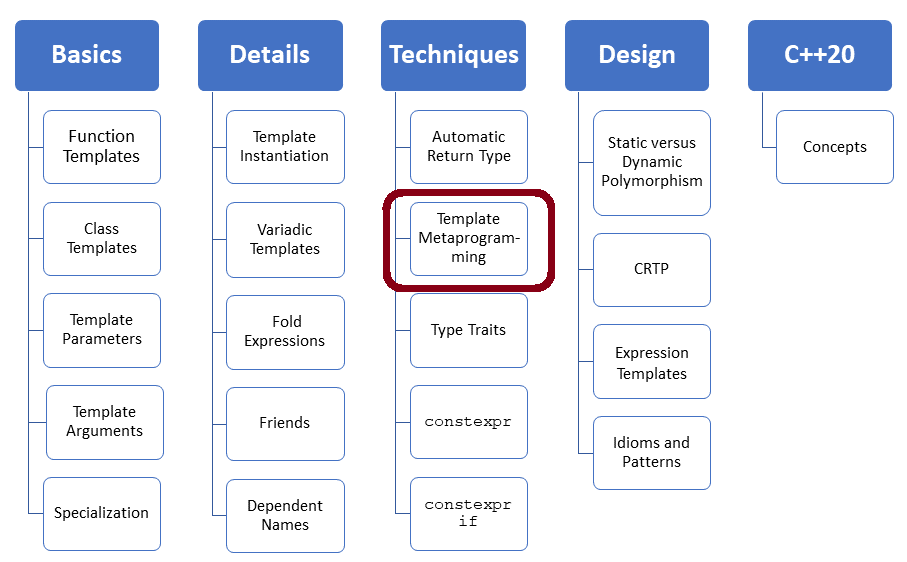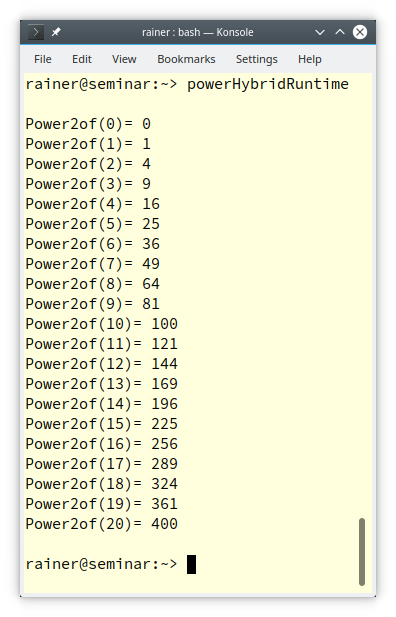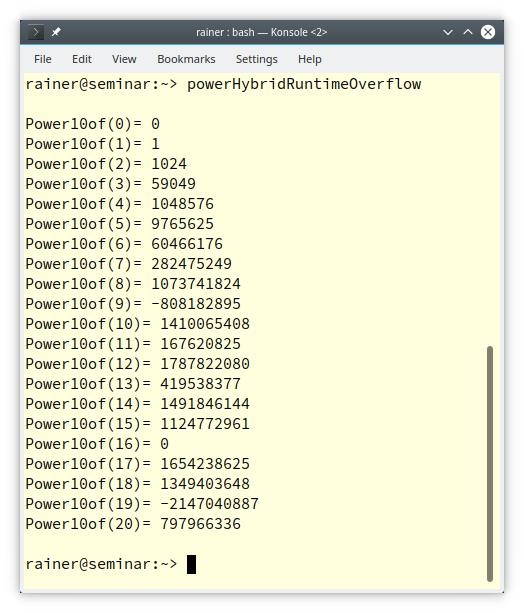# Template Metaprogramming – Hybrid Programming

First of all, hybrid programming is not an official term. I created it to emphasize an exciting aspect of templates. The difference between function arguments and template arguments.I ended my last post, “Template Metaprogramming – How it Works” with a riddle. Here is the context for the riddle.

## The Riddle

The function `power` and `Power` calculate the pow(2, 10). `power` is executed at run time and `Power` at compile time.

```// power.cpp

#include <iostream>

int power(int m, int n) {
int r = 1;
for(int k = 1; k <= n; ++k) r *= m;
return r;
}

template<int m, int n>
struct Power {
static int const value = m * Power<m, n-1>::value;
};

template<int m>
struct Power<m, 0> {
static int const value = 1;
};

int main() {

std::cout << '\n';

std::cout << "power(2, 10)= " << power(2, 10) << '\n';
std::cout << "Power<2,10>::value= " << Power<2, 10>::value << '\n';

std::cout << '\n';
}
```

If you want more details about both functions, read my previous post, “Template Metaprogramming – How it Works“.

##Modernes C++ Mentoring

Be part of my mentoring programs:

• "Fundamentals for C++ Professionals" (open)
• "Design Patterns and Architectural Patterns with C++" (open)
• "C++20: Get the Details" (reopens December 2023)
• Do you want to stay informed about my mentoring programs: Subscribe via E-Mail.

So far, so good, but what is happening in the following example?

```// powerHybrid.cpp

#include <iostream>

template<int n>
int Power(int m){
return m * Power<n-1>(m);
}

template<>
int Power<0>(int m){
return 1;
}

int main() {

std::cout << '\n';

std::cout << "Power<0>(10): " << Power<0>(20) << '\n';
std::cout << "Power<1>(10): " << Power<1>(10) << '\n';
std::cout << "Power<2>(10): " << Power<2>(10) << '\n';

std::cout << '\n';

}```

As expected, `Power` does its job.Here is the riddle in short one more: Is `Power` a function or a metafunction?

## Hybrid Programming

To make it short.

The calls` Power<0>(10)`,` Power<1>(10)`, and `Power<2>(10)` use sharp and round brackets and calculate 10 to the power of 0, 1, and 2. This means 0, 1, and 2 are compile-time arguments, and 10 is a run-time argument. To say it differently: Power is, at the same time, a function and a metafunction. Let me elaborate more on this point.

### Power at Run Time

First, I can instantiate `Power` for 2, give it the name` Power2` and use it in a for-loop.

```// powerHybridRuntime.cpp

#include <iostream>

template<int n>
int Power(int m){
return m * Power<n-1>(m);
}

template<>
int Power<0>(int m){
return 1;
}

int main() {

std::cout << '\n';

auto Power2of = Power<2>;

for (int i = 0; i <= 20; ++i) {
std::cout << "Power2of(" << i << ")= "
<< Power2of(i)  << '\n';
}

std::cout << '\n';

}```

`Power2o`f enables it to calculate the squares of 0 … 20 at run time.You cannot invoke `Power` with different template arguments in the for-loop. Template instantiation requires a constant expression. To make it short: The following use of Power fails with a compile-time error that “`the value of 'i' is not usable in a constant expression`“.

for (int i = 0; i <= 20; ++i) {

std::cout << “Power<“ << i << “>(2)= “ << Power<i>(2) << ‘\n’;

}

Honestly, there is a more interesting difference between a function and a metafunction.

### Power at Compile Time

When you study the previous program `powerHybrid.cpp` in C++ Insights, you see that each usage of Power with a different template argument creates a new type.

This means that the invocation ` Power<2>(10)` causes the recursive template instantiation for `Power<1>(10)`, and `Power<0>(10)`. Here is the output of C++ Insights.To sum up my observation. Each template instantiation creates a new type.

### Creating New Types

When you use a template such as `Power`, `std::vector`, or `std::array`, you can invoke it with two kinds of arguments: function arguments and template arguments. The function arguments go into the round brackets (`( ... )`) and the template arguments go into the sharp brackets (`<...>`). The template arguments create new types. Or, to put it the other way around. You can parameterize templates in two ways: at compile time with sharp brackets (`<...>`). and at run time with round brackets (`( ... )`.

```auto res1 = Power<2>(10);                       // (1)
auto res2 = Power<2>(11);                       // (2)
auto rest3 = Power<3>(10);                      // (3)

std::vector<int> myVec1(10);                    // (1)
std::vector<int> myVec2(10, 5);                 // (2)
std::vector<double> myDouble(5);                // (3)

std::array<int, 3> myArray1{ 1, 2, 3};          // (1)
std::array<int, 3> myArray2{ 1, 2, 3};          // (2)
std::array<double, 3> myArray3{ 1.1, 2.2, 3.3}; // (3)
```

• (1) creates a new `Power` instance,` std::vector` of length 10, or a `std::array` with three elements
• (2) reuses the already created types in the previous lines (1)
• (3) creates a new type

A few of my German readers already pointed it out. My metafunction Power has a significant flaw.

### The Big Flaw

When I instantiate `Power` with a negative or too-big number, I get undefined behavior.

1. `Power<-1>(10)` causes an infinite template instantiation because the boundary condition Power<0>(10) does not apply.
2. `Power<200>(10)` causes an `int` overflow.

The first issues can be fixed by using a` static_assert` inside the `Power` template:` static_assert(n >= 0, "exponent must be >= 0");. `There is no simple solution for the second issue.

```// powerHybridRuntimeOverflow.cpp

#include <iostream>

template<int n>
int Power(int m){
return m * Power<n-1>(m);
}

template<>
int Power<0>(int m){
return 1;
}

int main() {

std::cout << '\n';

auto Power10of = Power<10>;

for (int i = 0; i <= 20; ++i) {
std::cout << "Power10of(" << i << ")= "
<< Power10of(i)  << '\n';
}

std::cout << '\n';

}
```

The overflow starts with` Power10of(9). pow(9, 10) is `3,486,784,40

` `## My Disclaimer

At the end of these three posts, “Template Metaprogramming – How it All Started“, “Template Metaprogramming – How it Works” about template metaprogramming, I have to make a disclaimer. I don’t want that you use templates to program at compile time. Most of the time, `constexpr` (C++11) or `consteval` (C++20 is the better choice.

I explained template metaprogramming for two reasons.

1. Template metaprogramming helps you better understand templates and the process of template instantiation.
2. The type-traits library applies the idea and uses the conventions of template metaprogramming.

## What’s next?

In my next post, I will write about the type-traits library.  The type-traits library (C++11) is template metaprogramming in a beautiful guise.

Thanks a lot to my Patreon Supporters: Matt Braun, Roman Postanciuc, Tobias Zindl, G Prvulovic, Reinhold Dröge, Abernitzke, Frank Grimm, Sakib, Broeserl, António Pina, Sergey Agafyin, Андрей Бурмистров, Jake, GS, Lawton Shoemake, Jozo Leko, John Breland, Venkat Nandam, Jose Francisco, Douglas Tinkham, Kuchlong Kuchlong, Robert Blanch, Truels Wissneth, Kris Kafka, Mario Luoni, Friedrich Huber, lennonli, Pramod Tikare Muralidhara, Peter Ware, Daniel Hufschläger, Alessandro Pezzato, Bob Perry, Satish Vangipuram, Andi Ireland, Richard Ohnemus, Michael Dunsky, Leo Goodstadt, John Wiederhirn, Yacob Cohen-Arazi, Florian Tischler, Robin Furness, Michael Young, Holger Detering, Bernd Mühlhaus, Matthieu Bolt, Stephen Kelley, Kyle Dean, Tusar Palauri, Dmitry Farberov, Juan Dent, George Liao, Daniel Ceperley, Jon T Hess, Stephen Totten, Wolfgang Fütterer, Matthias Grün, Phillip Diekmann, Ben Atakora, Ann Shatoff, Rob North, Bhavith C Achar, and Marco Parri Empoli.

Thanks, in particular, to Jon Hess, Lakshman, Christian Wittenhorst, Sherhy Pyton, Dendi Suhubdy, Sudhakar Belagurusamy, Richard Sargeant, Rusty Fleming, John Nebel, Mipko, Alicja Kaminska, Slavko Radman, and David Poole.

 My special thanks to EmbarcaderoMy special thanks to PVS-StudioMy special thanks to Tipi.buildMy special thanks to Take Up Code## Seminars

I’m happy to give online seminars or face-to-face seminars worldwide. Please call me if you have any questions.

### Standard Seminars (English/German)

Here is a compilation of my standard seminars. These seminars are only meant to give you a first orientation.

• C++ – The Core Language
• C++ – The Standard Library
• C++ – Compact
• C++11 and C++14
• Concurrency with Modern C++
• Design Pattern and Architectural Pattern with C++
• Embedded Programming with Modern C++
• Generic Programming (Templates) with C++

#### New

• Clean Code with Modern C++
• C++20

### Contact Me

Modernes C++ Mentoring,0 replies